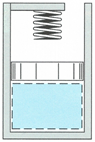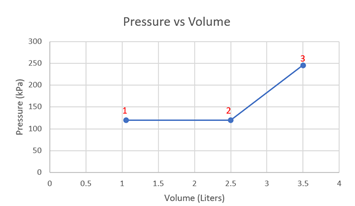## A Piston Problem Twist

Consider the cylinder system shown in the figure below, which contains 1.5 g of air initially at 20°C and 120 kPa. At constant pressure, heat is then added to the air, raising the piston. The piston touches the spring when the air occupies a 2.5 liter volume. Heat in continually added until the air reaches a volume of 3.5 liters and a 2000K temperature. The universal gas constant is R(u) = 8.314 kJ/(kmol∙K) and the molar mass of air is 29 kg/kmol.
1. How much work is done by the air?
2. Sketch the process on a Pressure vs Volume diagram, labeling all the important states.Hint
$$V=\frac{mRT}{P}$$$where $$V$$ is volume, $$m$$ is mass, $$R$$ is the universal gas constant, $$T$$ is temperature, and $$P$$ is pressure. Hint 2 $$W=P(V_2-V_1)$$$
$$W=\frac{1}{2}(P_1+P_2)(V_2-V_1)$$$where $$W$$ is work, $$P$$ is pressure, and $$V$$ is volume. $$V_1=\frac{mRT_1}{P_1}=\frac{(1.5\cdot 10^{-3})(\frac{8.314}{29})(293)}{120}=1.05\:L$$$
where $$V$$ is volume, $$m$$ is mass, $$R$$ is the gas constant, $$T$$ is temperature, and $$P$$ is pressure.
$$P_3=\frac{mRT_3}{V_3}=\frac{(1.5\cdot 10^{-3})(\frac{8.314}{29})(2000)}{3.5\cdot 10^{-3}}=246\: kPa$$$Solving for work, $$W_{1-2}=P_1(V_2-V_1)=120(2.5-1.05)=174\: J$$$
$$W_{2-3}=\frac{1}{2}(P_2+P_3)(V_3-V_2)=\frac{1}{2}(120+246)(3.5-2.5)=183\: J$$$$$W=W_{1-2}+W_{2-3}=174+183=357\:J$$$
Pressure vs Volume diagram,1. 357 J